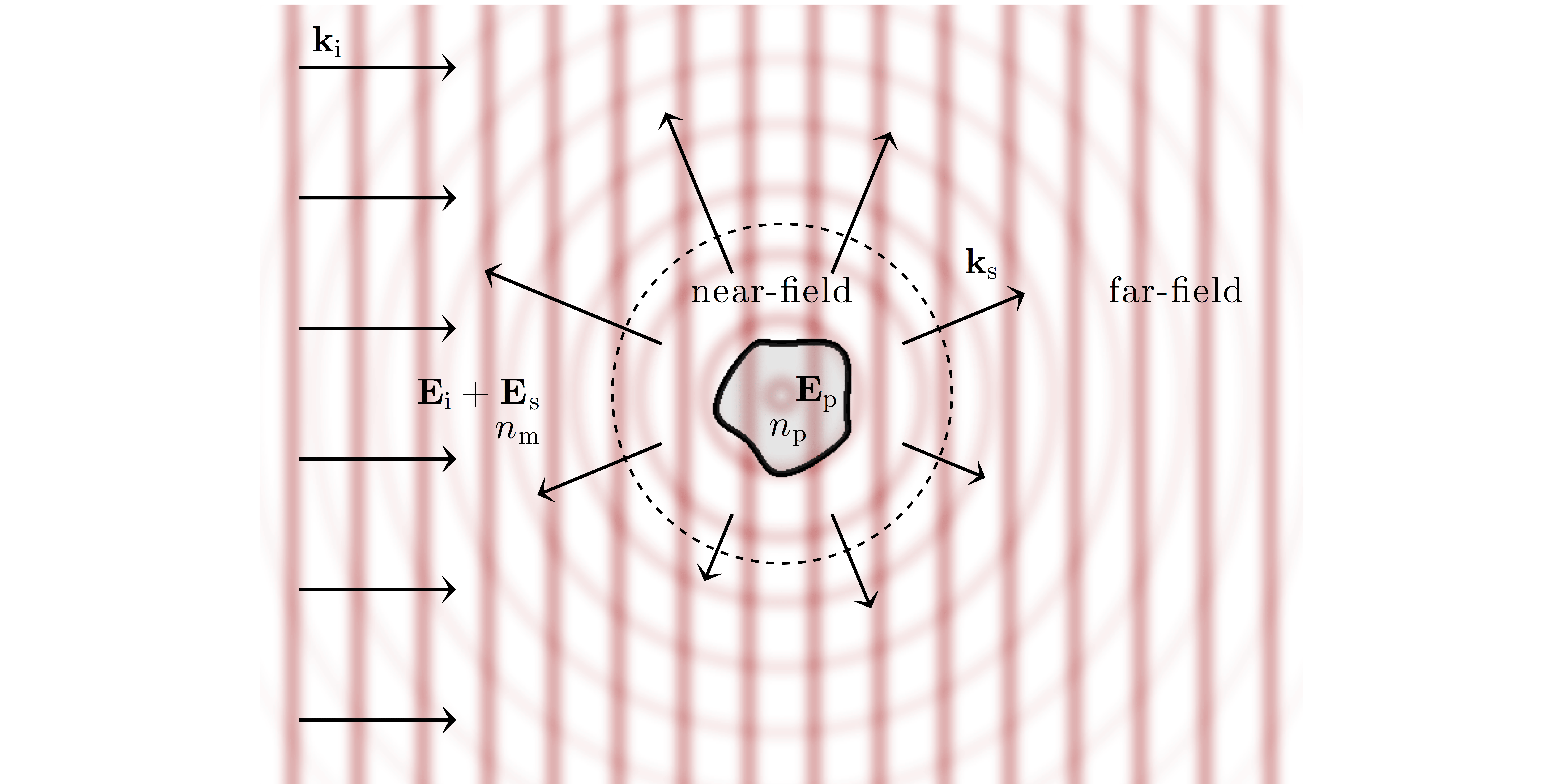# Chapter 5 — Electromagnetic Theory

So far we have described optical forces and torques in two extreme regimes, where their expression is greatly simplified: for particles either much larger (ray optics regime, Chapter 2) or much smaller (Rayleigh regime, Chapter 3) than the wavelength of the trapping light. It is now time to turn our attention to the intermediate regime, where, as is often the case in experiments, the particle dimensions are com- parable to the wavelength. Here, the previously used approximations break down and a complete wave-optical modelling of the particle-light interaction based on electromagnetic scattering theory is necessary in order to obtain accurate results, as shown in Fig. 5.1. In this Chapter, we set out the general equations describing optical forces and torques within the framework of electromagnetic scattering theory. We start by discussing the general scattering problem and the Maxwell stress tensor, which describes the mechanical interaction of light with matter. Then, we derive the optical force and torque exerted by a plane wave in terms of the Maxwell stress tensor. Finally, we discuss optical trapping, where the incident light is focused through an objective lens, by expanding the optical fields using the angular spec- trum representation described in Chapter 4. We will leave the detailed discussion of the numerical methods practically used to compute optical forces for complex particles to Chapter 6.

5  Electromagnetic theory

5.1  Conservation laws and the Maxwell stress tensor
5.1.1  Angular momentum of light

5.2  Light scattering
5.2.1  Solution of the Helmholtz equation
5.2.2  The scattering problem
5.2.3  Multipole expansion
5.2.4  Transition matrix
5.2.5  Mie scattering

5.3  Optical force and torque
5.3.1  Optical force
5.3.2  Optical torque

5.4  Optical force from a plane wave

5.5  Transfer of spin angular momentum to a sphere

5.6  Optical force in an optical tweezers
5.6.1  Orbital angular momentum

Problems

References

## 6 thoughts on “Chapter 5 — Electromagnetic Theory”

1.Bruno Fernando says:

Hey, do you have solutions to the exercises? I find it really hard to find help in other materials since different sources use different notations. It would be so nice to see a solution manual to the analytic problems.

1.giovannivolpe says:

Hi, Thank you for your feedback. Unfortunately, at the moment we don’t have the solutions typed in a readable format, but we are planning to have them some time in the future.

2.Bruno Fernando says:

Exercise 5.2.23, page 136: f is a vector, while the rhs is a scalar.

1.giovannivolpe says:

Yes, you are right. f is a scalar and is wrongly indicated as a vector. I’ve posted an erratum.

3.Bruno Fernando says:

Also, could someone please check the definitions of the vector spherical harmonics (5.76 page 127). I believe one of the unit vectors r should be the actual vector r.

1.giovannivolpe says:

Yes, thank you for this comment. We’ll post an erratum.